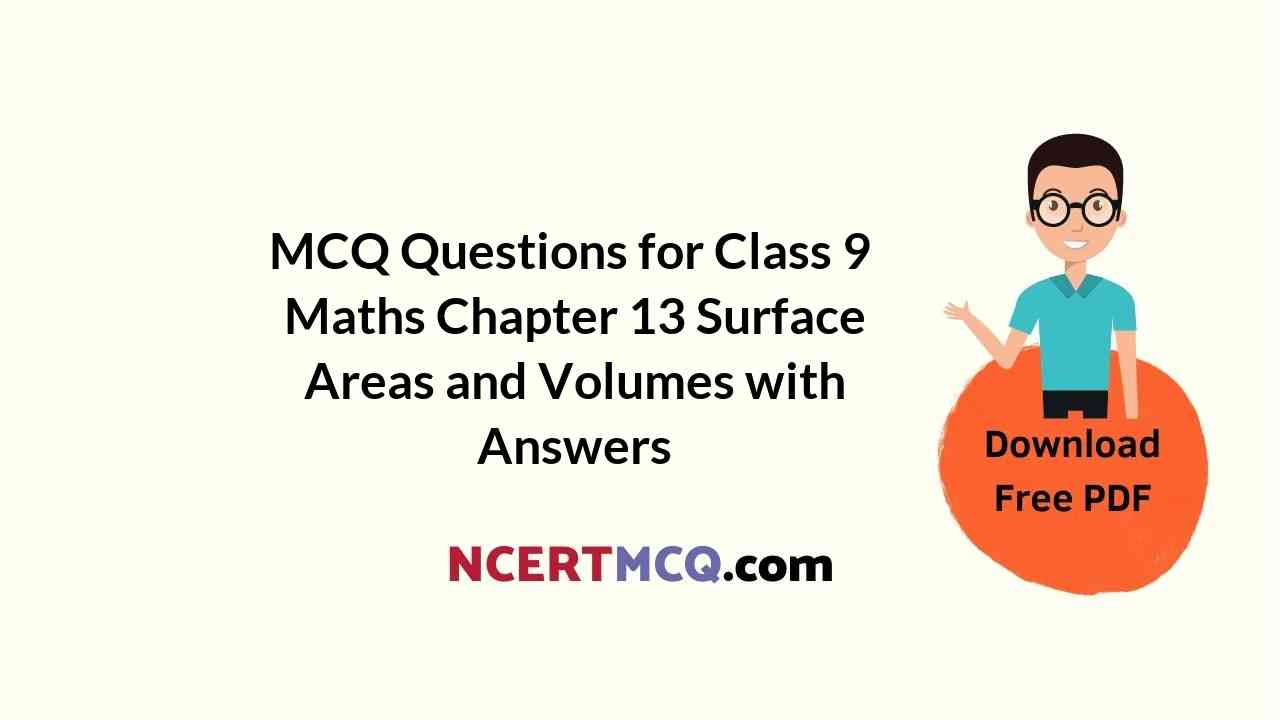Check the below Online Education NCERT MCQ Questions for Class 9 Maths Chapter 13 Surface Areas and Volumes with Answers Pdf free download. MCQ Questions for Class 9 Maths with Answers were prepared based on the latest exam pattern. We have provided Surface Areas and Volumes Class 10 Maths MCQs Questions with Answers to help students understand the concept very well. https://ncertmcq.com/mcq-questions-for-class-9-maths-with-answers/

Students can also refer to NCERT Solutions for Class 9 Maths Chapter 13 Surface Areas and Volumes for better exam preparation and score more marks.

## Online Education for Surface Areas and Volumes Class 9 MCQs Questions with Answers

Surface Area And Volume Class 9 MCQ Chapter 13 Question 1.
If the perimeter of one of the faces of a cube is 40 cm, then its volume is:
(a) 6000 cm³
(b) 1600 cm³
(c) 1000 cm³
(d) 600 cm³

Surface Area And Volume Class 9 MCQ With Answers Chapter 13 Question 2.
A cuboid having surface areas of 3 adjacent faces as a, b and c has the volume:
(a) 3$$\sqrt{abc}$$
(b) $$\sqrt{abc}$$
(c) abc
(d) (abc)²

Answer: (b) $$\sqrt{abc}$$

Class 9 Maths Chapter 13 MCQ Question 3.
The radius of a cylinder is doubled and the height remains the same. The ratio between the volumes of the new cylinder and the original cylinder is
(a) 1 : 2
(b) 3 : 1
(c) 4 : 1
(d) 1 : 8

MCQ Questions For Class 9 Maths Ncert Chapter 13 Question 4.
Length of diagonals of a cube of side a cm is
(a) √2a cm
(b) √3a cm
(c) $$\sqrt{3a}$$ cm
(d) 1 cm

Ch 13 Maths Class 9 MCQ  Question 5.
Volume of spherical shell is
(a) $$\frac{2}{3}$$ πr³
(b) $$\frac{3}{4}$$ πr³
(c) $$\frac{4}{3}$$ π(R³ – r³)
(d) None of these

Answer: (c) $$\frac{4}{3}$$ π(R³ – r³)

Class 9 Surface Area And Volume MCQ Chapter 13 Question 6.
Volume of hollow cylinder
(a) π(R² – r²)h
(b) πR²h
(c) πr²h
(d) πr²(h1 – h1)

Class 9 Maths Ch 13 MCQ Question 7.
The radius of a sphere is 2r, then its volume will be
(a) $$\frac{4}{3}$$ πr³
(b) 4πr³
(c) $$\frac{8}{3}$$ πr³
(d) $$\frac{32}{3}$$ πr³

Answer: (d) $$\frac{32}{3}$$ πr³

MCQ On Surface Area And Volume Class 9 Pdf Chapter 13 Question 8.
In a cylinder, radius is doubled and height is halved, curved surface area will be
(a) halved
(b) doubled
(c) same
(d) four time

MCQ Questions For Class 9 Maths Chapter 13 Question 9.
The total surface area of a cone whose radius is $$\frac{r}{2}$$ and slant height 2l is
(a) 2πr(l + r)
(b) πr(l + $$\frac{r}{4}$$)
(c) πr(l + r)
(d) 2πrl

Answer: (b) πr(l + $$\frac{r}{4}$$)

Surface Area And Volume MCQ Class 9 Chapter 13 Question 10.
The radius of a hemispherical balloon increases from 6 cm to 12 cm as air is being pumped into it. The ratios of the surface areas of the balloon in the two cases is
(a) 1 : 4
(b) 1 : 3
(c) 2 : 3
(d) 2 : 1

Chapter 13 Maths Class 9 MCQ Question 11.
The length of the longest pole that can be put in a room of dimension (10 m × 10 m × 5 m) is
(a) 15 m
(b) 16 m
(c) 10 m
(d) 12 m

MCQ Questions For Class 9 Maths Surface Area And Volume Chapter 13 Question 12.
The lateral surface area of a cube is 256 m³. The volume of the cube is
(a) 512 m³
(b) 64 m³
(c) 216 m³
(d) 256 m³

MCQ Of Surface Area And Volume Class 9 Chapter 13 Question 13.
The radii of two cylinders are in the ratio of 2 : 3 and their heights are in the ratio of 5 : 3. The ratio of their volumes is
(a) 10 : 17
(b) 20 : 27
(c) 17 : 27
(d) 20 : 37

Class 9 Maths Chapter 13 MCQ With Answers Question 14.
A cone is 8.4 cm high and the radius of its base is 2.1 cm. It is melted and recast into a shape. The radius of the sphere is
(a) 4.2 cm
(b) 2.1 cm
(c) 2.4 cm
(d) 1.6 cm

MCQ On Surface Area And Volume Class 9 Chapter 13 Question 15.
The total surface area of a cube is 96 cm². The volume of the cube is
(a) 8 cm³
(b) 512 cm³
(c) 64 cm³
(d) 27 cm³